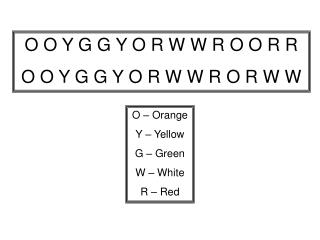DownloadDownload PresentationO O Y G G Y O R W W R O O R R O O Y G G Y O R W W R O R W W

O O Y G G Y O R W W R O O R R O O Y G G Y O R W W R O R W W

Télécharger la présentationO O Y G G Y O R W W R O O R R O O Y G G Y O R W W R O R W W

- - - - - - - - - - - - - - - - - - - - - - - - - - - E N D - - - - - - - - - - - - - - - - - - - - - - - - - - -
Presentation Transcript

1. O O Y G G Y O R W W R O O R R O O Y G G Y O R W W R O R W W O – Orange Y – Yellow G – Green W – White R – Red

2. O – Orange Y – Yellow G – Green W – White R – Red B – Blue W W G G B B G Y Y O O R R W G G Y Y O O R G G Y Y O O R W W G G B B G Y Y O O R R W

3. P P P P P P P R G B P W W W W W P P P P P B B P B R P – Purple W – White R – Red B – Blue G - Green

4. O – Orange Y – Yellow G – Green W – White R – Red B – Blue P – Purple Bl - Black O O G O O G O G Bl P B B G R O Y R R O Y R Y P B G P Bl W W Bl B Y G O W Y G B O G W W Bl B Y G O W Y G Y O R W

5. O – Orange Y – Yellow G – Green W – White R – Red B – Blue P – Purple Bl - Black G W R O Y G W W B Y G B P Bl W W Y G Y O R O Y O R W R O R W R W

6. O – Orange Y – Yellow G – Green W – White R – Red W O Y G W O Y G W O Y G Y W O R O O R W W O G G G Y O Y G Y W R W

7. Y – Yellow P - Purple B - Blue R – Red B Y R Y B B B Y Y Y B P P B Y R Y B B B B Y Y B Y R